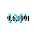### leetcode Question 100: Spiral Matrix I

Spiral Matrix I

Given a matrix of m x n elements (m rows, n columns), return all elements of the matrix in spiral order.
For example,
Given the following matrix:
[
[ 1, 2, 3 ],
[ 4, 5, 6 ],
[ 7, 8, 9 ]
]

You should return [1,2,3,6,9,8,7,4,5].

Analysis:

When I first face this problem, it took me hours to handle the array indices, and always missed some cases. Then I give up get a whole line once, but tried to get one element in every step. The problem became much easier and took me less than 10 minutes to solve it!

Let's think in this way:
There are m*n elements I need to push into the result vector. So we just do it one by one.
For each element, there are only 4 directions can go to the next, left, down, right, and up.
The conditions are almost the same: while the next element in such direction is available, go to the next. Otherwise try the next one direction.
Available above means: (1) meet the bound of the array. (2) The next element has been visited.

Once we get the algorithm, coding becomes pretty clear, just use a mask bool array to store the visited elements. Big loop from 1 to n*m, (i,j) is the current position, keep try 4 directions, until all the elements are popped.

Code:
class Solution {
public:
vector<int> spiralOrder(vector<vector<int> > &matrix) {
// Start typing your C/C++ solution below
// DO NOT write int main() function
vector<int> res;
if (matrix.empty()){return res;}
if (matrix.size()==1){return matrix;}
int m = matrix.size();
int n = matrix.size();
int i=0;
int j=0;
int k=0;
res.push_back(matrix[i][j]);
while (k<m*n-1){
j++;
k++;
res.push_back(matrix[i][j]);
}

i++;
k++;
res.push_back(matrix[i][j]);
}

j--;
k++;
res.push_back(matrix[i][j]);
}

i--;
k++;
res.push_back(matrix[i][j]);
}
}
return res;
}
};


1.Might be challenge in interview though. Since there is a solution that requires only constant space. :)

2.here is my code I think simpler than that :-)

class Solution {
public:
vector v;
vector spiralOrder(vector > &matrix)
{
int n=matrix.size(),m;
if(n!=0)
{
m=matrix.size();
spiral(matrix,0,n-1,0,m-1);
}
return v;
}
void spiral(vector > v1,int r1,int r2,int c1,int c2)
{
int i;
if(r1==r2)
{
for(i=c1;i<=c2;i++)
v.push_back(v1[r1][i]);
}
else if(c1==c2)
{
for(i=r1;i<=r2;i++)
v.push_back(v1[i][c1]);
}
else if(r1<=r2&&c1<=c2)
{
for(i=c1;i<=c2;i++)
v.push_back(v1[r1][i]);
for(i=r1+1;i<=r2;i++)
v.push_back(v1[i][c2]);
for(i=c2-1;i>=c1;i--)
v.push_back(v1[r2][i]);
for(i=r2-1;i>=r1+1;i--)
v.push_back(v1[i][c1]);
spiral(v1,r1+1,r2-1,c1+1,c2-1);
}
else
return ;
}
};

3.http://computerarea96.blogspot.in/

4.This comment has been removed by the author.